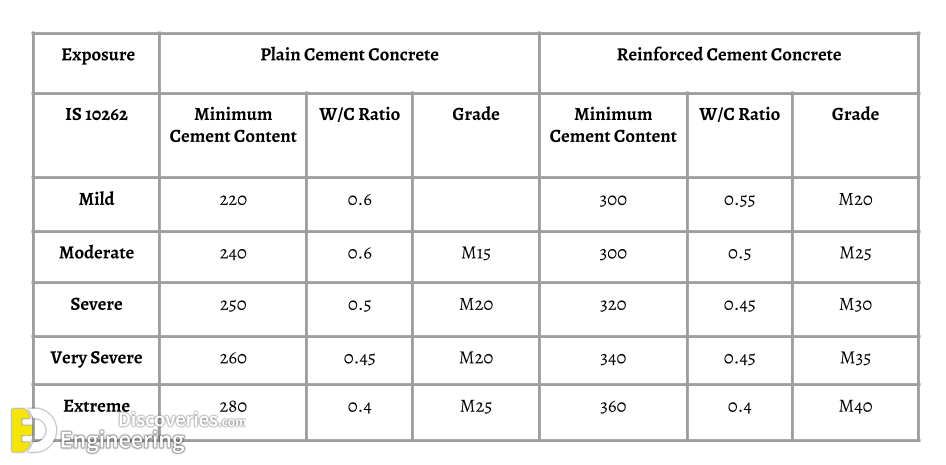### WHAT IS WATER CEMENT RATIO? HOW TO CALCULATE WATER CEMENT RATIO.

Water-Cement Ratio is defined as the ratio between the weight of the water to the weight of cement.
In general terms, w/c ratio means how much water it requires for the particular mix. We all know that the water-cement ratio will directly affect the strength of concrete. The strength of concrete either increases or decreases will depend upon the w/c ratio. It can also affect the workability of concrete, which is most important to concreting process enjoy mixing, placing and compacting. Therefore, it’s most important to calculate the water-cement ratio while mixing design. As this is very important for both fresh and hardened concrete.Water-cement ratio table

Here we list the water-cement ratio table as per IS code 10262How to calculate the quantity of water for Concrete?

Normally, the water-cement ratio is between 0.4 to 0.6 as per IS standard.

We take 0.42 as a ratio of W/C.

Quantity of water = 0.42 x 50  = 21 Liters (1 bag cement = 50 Kg)

So the required quantity of water is 21 Liters per cement bag.

We shall interpret the water-cement ratio by Thumb Rule Calculation for M15 grade concrete.

M25 ( 1 : 2.28 : 3.27)

Cement : 350 Kg/ M3

20 mm Jelly : 670 Kg/ M3

12.5 mm Jelly : 445 Kg/ M3

River sand : 775 Kg/ M3

Total = 350 + 670 + 445 + 775 =  2240

Unit Weight of Water = 1000 Kg

Water ratio = 1000/2240 = 0.45.